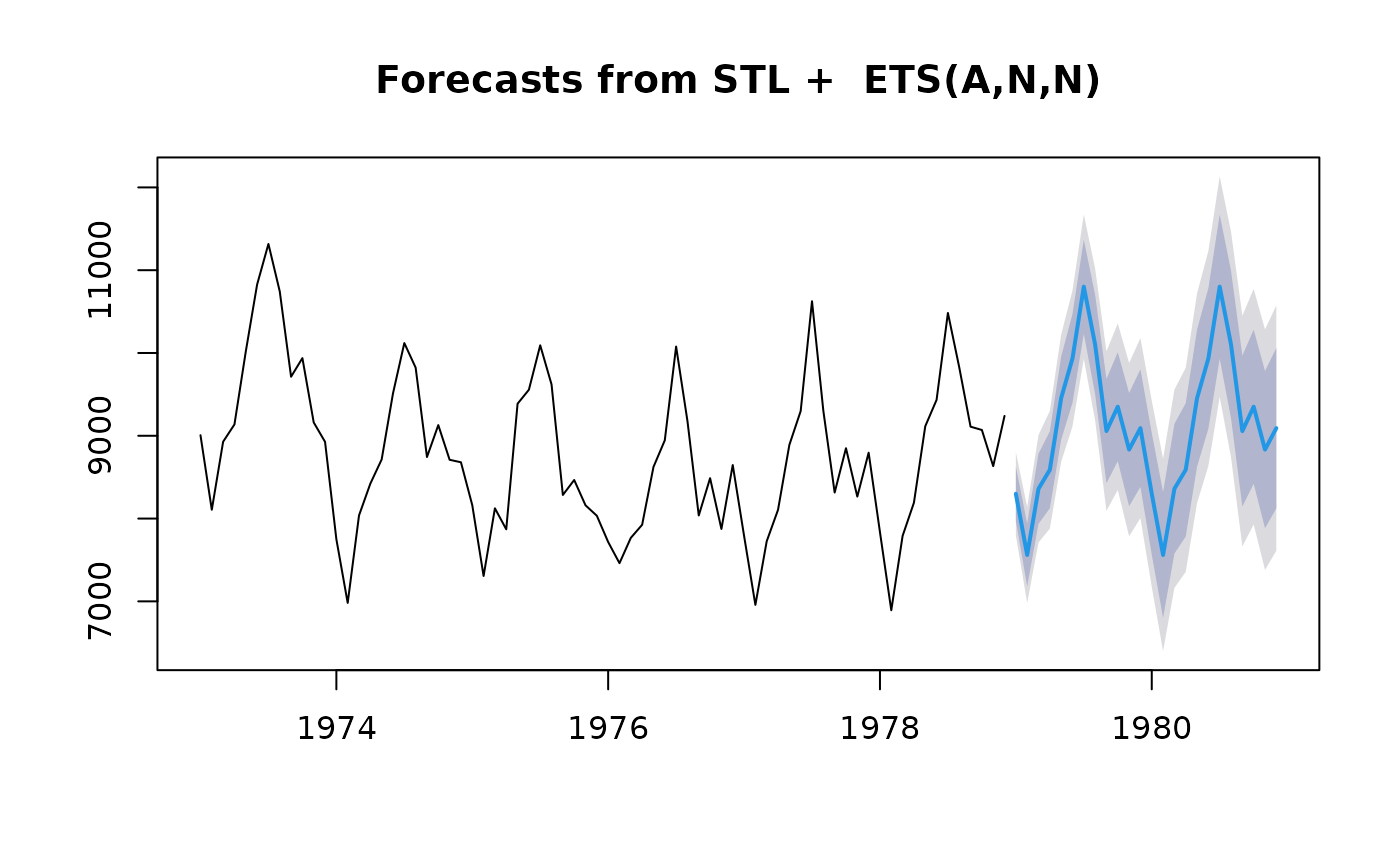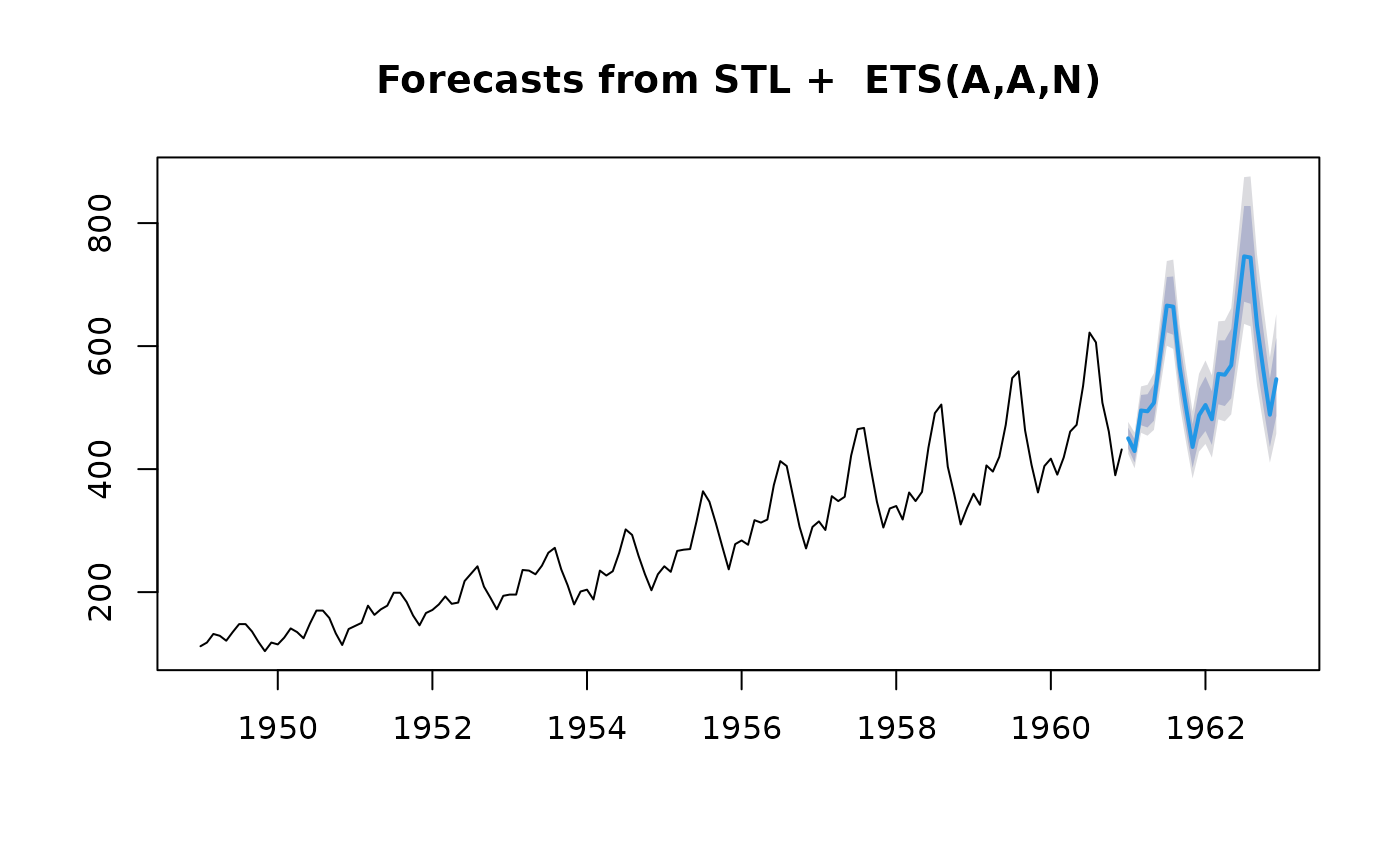Forecasts of STL objects are obtained by applying a non-seasonal forecasting method to the seasonally adjusted data and re-seasonalizing using the last year of the seasonal component.

## Usage

# S3 method for stl
forecast(
object,
method = c("ets", "arima", "naive", "rwdrift"),
etsmodel = "ZZN",
forecastfunction = NULL,
h = frequency(object$time.series) * 2, level = c(80, 95), fan = FALSE, lambda = NULL, biasadj = NULL, xreg = NULL, newxreg = NULL, allow.multiplicative.trend = FALSE, ... ) stlm( y, s.window = 7 + 4 * seq(6), robust = FALSE, method = c("ets", "arima"), modelfunction = NULL, model = NULL, etsmodel = "ZZN", lambda = NULL, biasadj = FALSE, xreg = NULL, allow.multiplicative.trend = FALSE, x = y, ... ) # S3 method for stlm forecast( object, h = 2 * object$m,
level = c(80, 95),
fan = FALSE,
lambda = object\$lambda,
newxreg = NULL,
allow.multiplicative.trend = FALSE,
...
)

stlf(
y,
h = frequency(x) * 2,
s.window = 7 + 4 * seq(6),
t.window = NULL,
robust = FALSE,
lambda = NULL,
x = y,
...
)

## Arguments

object

An object of class stl or stlm. Usually the result of a call to stl or stlm.

method

Method to use for forecasting the seasonally adjusted series.

etsmodel

The ets model specification passed to ets. By default it allows any non-seasonal model. If method!="ets", this argument is ignored.

forecastfunction

An alternative way of specifying the function for forecasting the seasonally adjusted series. If forecastfunction is not NULL, then method is ignored. Otherwise method is used to specify the forecasting method to be used.

h

Number of periods for forecasting.

level

Confidence level for prediction intervals.

fan

If TRUE, level is set to seq(51,99,by=3). This is suitable for fan plots.

lambda

Box-Cox transformation parameter. If lambda="auto", then a transformation is automatically selected using BoxCox.lambda. The transformation is ignored if NULL. Otherwise, data transformed before model is estimated.

Use adjusted back-transformed mean for Box-Cox transformations. If transformed data is used to produce forecasts and fitted values, a regular back transformation will result in median forecasts. If biasadj is TRUE, an adjustment will be made to produce mean forecasts and fitted values.

xreg

Historical regressors to be used in auto.arima() when method=="arima".

newxreg

Future regressors to be used in forecast.Arima().

allow.multiplicative.trend

If TRUE, then ETS models with multiplicative trends are allowed. Otherwise, only additive or no trend ETS models are permitted.

...

Other arguments passed to forecast.stl, modelfunction or forecastfunction.

y

A univariate numeric time series of class ts

s.window

Either the character string periodic'' or the span (in lags) of the loess window for seasonal extraction.

robust

If TRUE, robust fitting will used in the loess procedure within stl.

modelfunction

An alternative way of specifying the function for modelling the seasonally adjusted series. If modelfunction is not NULL, then method is ignored. Otherwise method is used to specify the time series model to be used.

model

Output from a previous call to stlm. If a stlm model is passed, this same model is fitted to y without re-estimating any parameters.

x

Deprecated. Included for backwards compatibility.

t.window

A number to control the smoothness of the trend. See stl for details.

## Value

stlm returns an object of class stlm. The other functions return objects of class forecast.

There are many methods for working with forecast objects including summary to obtain and print a summary of the results, while plot produces a plot of the forecasts and prediction intervals. The generic accessor functions fitted.values and residuals extract useful features.

## Details

stlm takes a time series y, applies an STL decomposition, and models the seasonally adjusted data using the model passed as modelfunction or specified using method. It returns an object that includes the original STL decomposition and a time series model fitted to the seasonally adjusted data. This object can be passed to the forecast.stlm for forecasting.

forecast.stlm forecasts the seasonally adjusted data, then re-seasonalizes the results by adding back the last year of the estimated seasonal component.

stlf combines stlm and forecast.stlm. It takes a ts argument, applies an STL decomposition, models the seasonally adjusted data, reseasonalizes, and returns the forecasts. However, it allows more general forecasting methods to be specified via forecastfunction.

forecast.stl is similar to stlf except that it takes the STL decomposition as the first argument, instead of the time series.

Note that the prediction intervals ignore the uncertainty associated with the seasonal component. They are computed using the prediction intervals from the seasonally adjusted series, which are then reseasonalized using the last year of the seasonal component. The uncertainty in the seasonal component is ignored.

The time series model for the seasonally adjusted data can be specified in stlm using either method or modelfunction. The method argument provides a shorthand way of specifying modelfunction for a few special cases. More generally, modelfunction can be any function with first argument a ts object, that returns an object that can be passed to forecast. For example, forecastfunction=ar uses the ar function for modelling the seasonally adjusted series.

The forecasting method for the seasonally adjusted data can be specified in stlf and forecast.stl using either method or forecastfunction. The method argument provides a shorthand way of specifying forecastfunction for a few special cases. More generally, forecastfunction can be any function with first argument a ts object, and other h and level, which returns an object of class forecast. For example, forecastfunction=thetaf uses the thetaf function for forecasting the seasonally adjusted series.

stl, forecast.ets, forecast.Arima.

Rob J Hyndman

## Examples


tsmod <- stlm(USAccDeaths, modelfunction = ar)
plot(forecast(tsmod, h = 36))decomp <- stl(USAccDeaths, s.window = "periodic")
plot(forecast(decomp))plot(stlf(AirPassengers, lambda = 0))